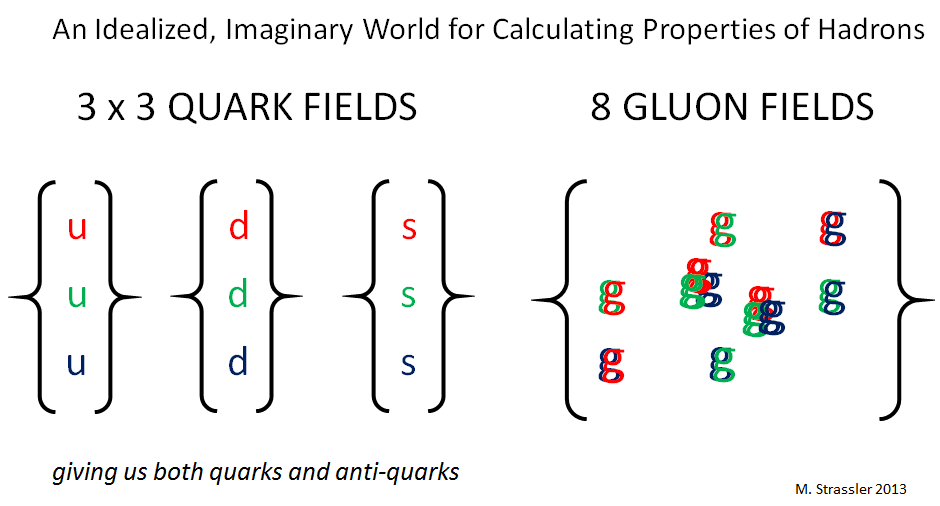## Quantum Field Theory, String Theory, and Predictions (Part 6)

For More Advanced Non-Experts

[This is part 6 of a series, which begins here.]

I’ve explained in earlier posts how we can calculate many things in the quantum field theory that is known as the “Standard Model” of particle physics, itself an amalgam of three, simpler quantum field theories.

When forces are “weak”, in the technical sense, calculations can generally be done by a method of successive approximation (called “perturbation theory”).  When forces are very “strong”, however, this method doesn’t work. Specifically, for processes involving the strong nuclear force, in which the distances involved are larger than a proton and the energies smaller than the mass-energy of a proton, some other method is needed.  (See Figure 1 of Part 5.)

One class of methods involves directly simulating, using a computer, the behavior of the quantum field theory equations for the strong nuclear force. More precisely, we simulate in a simplified version of the real world, the imaginary world shown in Figure 1 below, where

• the weak nuclear force and the electromagnetic force are turned off,
• the electron, muon, tau, neutrinos, W, Z and Higgs particles are ignored
• the three heavier types of quarks are also ignored

(See Figure 4 of Part 4 for more details.)  This makes the calculations a lot simpler.  And their results allow us, for instance, to understand why quarks and anti-quarks and gluons form the more complex particles called hadrons, of which protons and neutrons are just a couple of examples. Unfortunately, computer simulations still are nowhere near powerful enough for the calculation of some of the most interesting processes in nature… and won’t be for a long time.Fig 1: The idealized, imaginary world whose quantum field theory is used to make computer simulations of the real-world strong-nuclear force.

Another method I mentioned involves the use of an effective quantum field theory which describes the “objects” that the original theory produces at low energy. But that only works if you know what those objects are; in the real world [and the similar imaginary world of Figure 1] we know from experiment that those objects are pions and other low-mass hadrons, but generally we don’t know what they are.

This brings us to today’s story.  Our success with the Standard Model might give you the impression that we basically understand quantum field theory and how to make predictions using it, with a few exceptions. But this would be far, far from the truth. As far as we can tell, much (if not most) of quantum field theory remains deeply mysterious.